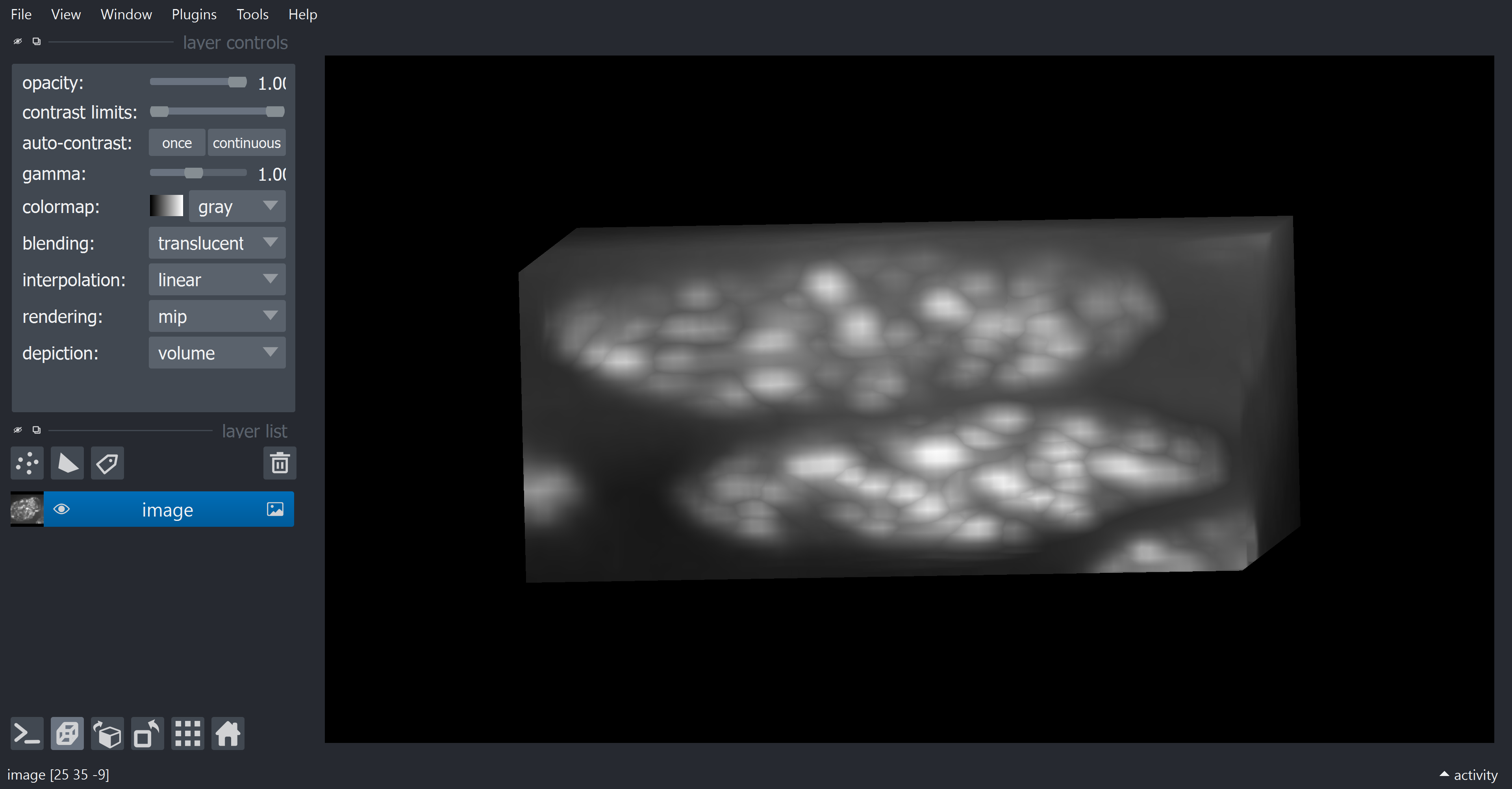# 3D Image Filters#

Image segmentation in 3D is challenging for several reasons. One typical problem is anisotropy, where voxel x and y sizes are not the same as their z size. Depending on the applied algorithms and respective given parameters, this may be a problem. Some algorithms only work for isotropic data.

from skimage.io import imread
from skimage import filters
import matplotlib.pyplot as plt

import napari
from napari.utils import nbscreenshot

# For 3D processing, powerful graphics
# processing units might be necessary
import pyclesperanto_prototype as cle


To demonstrate the workflow, we’re using cropped and resampled image data from the Broad Bio Image Challenge: Ljosa V, Sokolnicki KL, Carpenter AE (2012). Annotated high-throughput microscopy image sets for validation. Nature Methods 9(7):637 / doi. PMID: 22743765 PMCID: PMC3627348. Available at http://dx.doi.org/10.1038/nmeth.2083

image = imread("../../data/BMP4blastocystC3-cropped_resampled_8bit_2nuclei.tif")

voxel_size_x = 0.202 # um
voxel_size_y = 0.202 # um
voxel_size_z = 1 # um


Let’s check its shape.

print('pixels (z, y, x) = ', image.shape)

pixels (z, y, x) =  (32, 61, 74)


## Displaying anisotropic images in napari#

viewer = napari.Viewer()

INFO:xmlschema:Resource 'XMLSchema.xsd' is already loaded

viewer.add_image(image)
# Turns on 3D visualization
viewer.dims.ndisplay = 3

INFO:OpenGL.acceleratesupport:No OpenGL_accelerate module loaded: No module named 'OpenGL_accelerate'


If we rotate a view a bit in napari, we can see two nuclei. It also looks that the image is a bit squashed.

That’s because napari received raw image as a layer. Without any voxel size information, it assumes they are isotropic and this is the effect.

nbscreenshot(viewer)We would have to re-scale in each dimension to fix that. This can be done by calculating a ratio of sizes. Let’s use the x voxel size as a reference and calculate scaling fators for each dimension.

reference_size = voxel_size_x

factor_z = voxel_size_z / reference_size
factor_y = voxel_size_y / reference_size
factor_x = voxel_size_x / reference_size

print(factor_z, factor_y, factor_x)

4.9504950495049505 1.0 1.0


We can provide these factors to napari as a scaling factor. This way, napari can display the image as it is physically supposed toi be. We can do that by means of the scale property of the layer.

viewer.layers['image'].scale = [factor_z, factor_y, factor_x] # Z, Y, X order


Now, if we unzoom a bit your image in napari, it should look correct.

nbscreenshot(viewer)